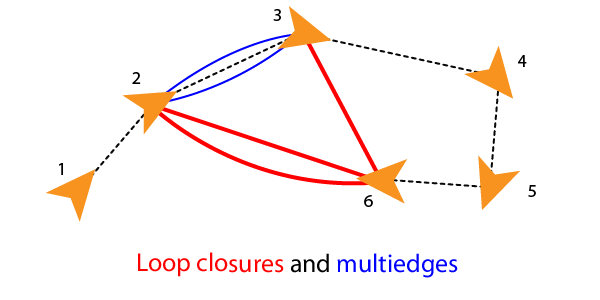poseGraph

Create 2-D pose graph

Description

A poseGraph object stores information for a 2-D pose graph representation. A pose graph contains nodes connected by edges. Each node estimate is connected to the graph by edge constraints that define the relative pose between nodes and the uncertainty on that measurement.

To construct a pose graph iteratively, use the addRelativePose function to add relative pose estimates and connect them to an existing node with specified edge constraints. Pose nodes must be specified relative to a pose node. Specify the uncertainty of the measurement using an information matrix.Adding an edge between two nonsequential nodes creates a loop closure in the graph. Multiple edges or multiedges between node pairs are also supported, which includes loop closures. To add additional edge constraints or loop closures, specify the node IDs using the addRelativePose function. When optimizing the pose graph, the optimizePoseGraph function finds a solution to satisfy all these edge constraints.To add landmark point nodes, use the addPointLandmark function. This function specifies nodes as xy-points without orientation estimates. Landmarks must be specified relative to a pose node.The lidarSLAM object performs lidar-based simultaneous localization and mapping, which is based around the optimization of a 2-D pose graph.

For 3-D pose graphs, see the poseGraph3D object or the Landmark SLAM Using AprilTag Markers example.

Creation

Description

example

poseGraph = poseGraph creates a 2-D pose graph object. Add poses using addRelativePose to construct a pose graph iteratively.

poseGraph = poseGraph('MaxNumEdges',maxEdges,'MaxNumNodes',maxNodes) specifies an upper bound on the number of edges and nodes allowed in the pose graph when generating code. This syntax is only required when generating code.

Properties

expand all

Number of nodes in pose graph, specified as a positive integer. Each node represents a pose measurement or a point landmark measurement. To specify relative poses between nodes, use addRelativePose. To specify a landmark pose, use addLandmarkPose. To get a list of all nodes, use edgeNodePairs.

Number of edges in pose graph, specified as a nonnegative integer. Each edge connects two nodes in the pose graph. Loop closure edges and landmark edges are included.

Number of loop closures in pose graph, specified as a nonnegative integer. To get the edge IDs of the loop closures, use the LoopClosureEdgeIDs property.

Loop closure edges IDs, specified as a vector of edge IDs.

Landmark node IDs, specified as a vector of IDs for each node.

Object Functions

 addPointLandmark Add landmark point node to pose graph addRelativePose Add relative pose to pose graph copy Create copy of pose graph edgeNodePairs Edge node pairs in pose graph edgeConstraints Edge constraints in pose graph edgeResidualErrors Compute pose graph edge residual errors findEdgeID Find edge ID of edge nodeEstimates Poses of nodes in pose graph removeEdges Remove loop closure edges from graph show Plot pose graph

Examples

collapse all

Optimize a pose graph based on the nodes and edge constraints. The pose graph used in this example is from the Intel Research Lab Dataset and was generated from collecting wheel odometry and a laser range finder sensor information in an indoor lab.

Load the Intel data set that contains a 2-D pose graph. Inspect the poseGraph object to view the number of nodes and loop closures.

disp(pg)
poseGraph with properties:

NumNodes: 1228
NumEdges: 1483
NumLoopClosureEdges: 256
LoopClosureEdgeIDs: [1228 1229 1230 1231 1232 1233 1234 1235 1236 ... ]
LandmarkNodeIDs: [1x0 double]

Plot the pose graph with IDs off. Red lines indicate loop closures identified in the dataset.

show(pg,'IDs','off');
title('Original Pose Graph')Optimize the pose graph. Nodes are adjusted based on the edge constraints and loop closures. Plot the optimized pose graph to see the adjustment of the nodes with loop closures.

updatedPG = optimizePoseGraph(pg);
figure
show(updatedPG,'IDs','off');
title('Updated Pose Graph')Grisetti, G., R. Kummerle, C. Stachniss, and W. Burgard. "A Tutorial on Graph-Based SLAM." IEEE Intelligent Transportation Systems Magazine. Vol. 2, No. 4, 2010, pp. 31–43. doi:10.1109/mits.2010.939925.